# tbrf Introduction

#### 2019-11-15

The tbrf package aims to provide functions that return rolling or moving statistical functions based on a user specified temporal time windows (eg. 1-year, 6-months, 5-hours, etc.). This package differs from most time-series analysis packages in R that rely on applying functions to a specific number of observations.

## Introduction

Currently tbrf provides functions to calculate binomial probability, geometric mean, mean, median, standard deviation, and sum. There is also a function to apply abritary R functions. This vignette demonstrates how time-windows are applied to irregularly spaced data and each of the functions.

## Basic usage

tbrf requires an input dataframe with two variables. First, a column with times or date-times formatted as class “POSIXt” or “Date”. Second, a column of observed values to calculate the statistic on. The package includes a suitable sample dataset:

data("Dissolved_Oxygen")

#> # A tibble: 6 x 6
#>   Station_ID Date       Param_Code Param_Desc             Average_DO Min_DO
#>        <int> <date>     <chr>      <chr>                       <dbl>  <dbl>
#> 1      12515 2000-01-03 00300      OXYGEN, DISSOLVED (MG~       6.19   6.19
#> 2      12515 2000-03-14 00300      OXYGEN, DISSOLVED (MG~       6.7    6.7
#> 3      12515 2000-03-16 00300      OXYGEN, DISSOLVED (MG~       6.41   6.41
#> 4      12515 2000-05-03 00300      OXYGEN, DISSOLVED (MG~       4.42   4.42
#> 5      12515 2000-06-15 00300      OXYGEN, DISSOLVED (MG~       4.86   4.86
#> 6      12515 2000-07-11 00300      OXYGEN, DISSOLVED (MG~       4.48   4.48

Core functions include five arguments.

.tbl = dataframe used by the function
x = column containing the values to calculate the statistic on
tcolumn = formatted date-time or date column
unit = character indicating the time unit used, one of "years", "months", "weeks", "days", "hours", "minutes", "seconds"
n = numeric, indicating the window length

If we want a 10-year rolling mean for the Dissolved_Oxygen dataset:

tbr_mean(Dissolved_Oxygen, x = Average_DO,
tcolumn = Date, unit = "years", n = 10)
#> # A tibble: 236 x 9
#>    Station_ID Date       Param_Code Param_Desc Average_DO Min_DO  mean
#>         <int> <date>     <chr>      <chr>           <dbl>  <dbl> <dbl>
#>  1      12515 2000-01-03 00300      OXYGEN, D~       6.19   6.19 NA
#>  2      12515 2000-03-14 00300      OXYGEN, D~       6.7    6.7   6.73
#>  3      12517 2000-03-14 00300      OXYGEN, D~       7.3    7.3   6.73
#>  4      12515 2000-03-16 00300      OXYGEN, D~       6.41   6.41  6.65
#>  5      12515 2000-05-03 00300      OXYGEN, D~       4.42   4.42  6.20
#>  6      12517 2000-06-14 00300      OXYGEN, D~       5.74   5.74  6.13
#>  7      12515 2000-06-15 00300      OXYGEN, D~       4.86   4.86  5.95
#>  8      12515 2000-07-11 00300      OXYGEN, D~       4.48   4.48  5.76
#>  9      12515 2000-09-12 00300      OXYGEN, D~       5.64   5.64  5.75
#> 10      12517 2000-10-17 00300      OXYGEN, D~       7.96   7.96  5.97
#> # ... with 226 more rows, and 2 more variables: lwr_ci <lgl>, upr_ci <lgl>

We can use a tidy workflow:

Dissolved_Oxygen %>%
group_by(Station_ID) %>%
tbr_mean(Average_DO, Date, "years", 10)
#> # A tibble: 236 x 9
#>    Station_ID Date       Param_Code Param_Desc Average_DO Min_DO  mean
#>         <int> <date>     <chr>      <chr>           <dbl>  <dbl> <dbl>
#>  1      12515 2000-01-03 00300      OXYGEN, D~       6.19   6.19 NA
#>  2      12515 2000-03-14 00300      OXYGEN, D~       6.7    6.7   6.44
#>  3      12517 2000-03-14 00300      OXYGEN, D~       7.3    7.3  NA
#>  4      12515 2000-03-16 00300      OXYGEN, D~       6.41   6.41  6.43
#>  5      12515 2000-05-03 00300      OXYGEN, D~       4.42   4.42  5.93
#>  6      12517 2000-06-14 00300      OXYGEN, D~       5.74   5.74  6.52
#>  7      12515 2000-06-15 00300      OXYGEN, D~       4.86   4.86  5.72
#>  8      12515 2000-07-11 00300      OXYGEN, D~       4.48   4.48  5.51
#>  9      12515 2000-09-12 00300      OXYGEN, D~       5.64   5.64  5.53
#> 10      12517 2000-10-17 00300      OXYGEN, D~       7.96   7.96  7
#> # ... with 226 more rows, and 2 more variables: lwr_ci <lgl>, upr_ci <lgl>

## Time windows

Generate some sample data:

# Some sample data
df <- data_frame(date = sample(seq(as.Date('2000-01-01'),
as.Date('2005-12-30'), by = "day"), 25)) %>%
bind_rows(data.frame(date = sample(seq(as.Date('2009-01-01'),
as.Date('2011-12-30'), by = "day"), 25))) %>%
arrange(date) %>%
mutate(value = 1:50)
#> Warning: data_frame() is deprecated, use tibble().
#> This warning is displayed once per session.

We can visualize the data captured in each rolling time window using tbr_misc() and the base::length():

df %>%
tbr_misc(x = value, tcolumn = date, unit = "years", n = 5, func = length) %>%
ggplot() +
geom_point(aes(date, value)) +
geom_errorbarh(aes(xmin = min_date, xmax = max_date,
y = value, color = results)) +
scale_color_distiller(type = "seq", palette = "OrRd",
direction = 1) +
guides(color = guide_colorbar(title = "Number of samples")) +
theme(legend.position = "bottom") +
labs(x = "Sample Date", y = "Sample Value",
title = "Window length and n",
caption = "Lines depict width of samples included in the time window\nColors indicate number of samples in the time window")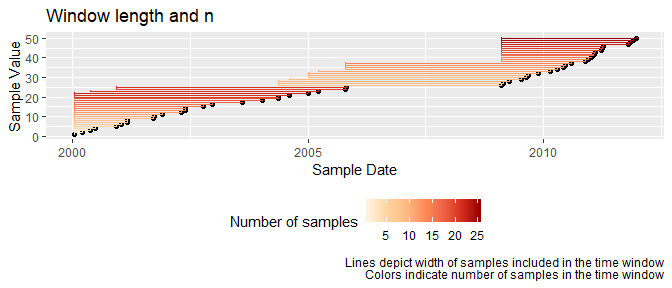## Examples

### Binomial Probability

Plot the binomial probability that dissolved oxygen fell below 5 mg/L during the previous 7-year period:

data("Dissolved_Oxygen")

Dissolved_Oxygen %>%
mutate(x = case_when(
Average_DO >= 5 ~ 0,
Average_DO < 5 ~ 1)) %>%
tbr_binom(x, Date, "years", 7, alpha = 0.05) %>%
ggplot() +
geom_line(aes(x = Date, y = PointEst)) +
geom_ribbon(aes(x = Date, ymin = Lower, ymax = Upper), alpha = 0.5)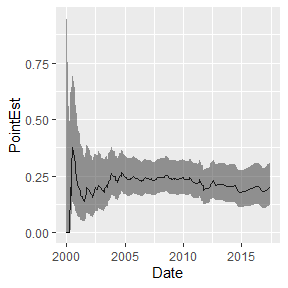### Geometric Mean

Plot the rolling 7-year geometric mean:

library(ggalt)

data_frame(date = sample(seq(as.Date('2001-01-01'),
as.Date('2017-12-31'),
by = "day"),
60),
x = rexp(60, 1/1000)) %>%
tbr_gmean(x, date, "years", 7, conf = 0.95, type = "perc") %>%
ggplot() +
geom_point(aes(date, x), alpha = 0.5) +
geom_step(aes(date, mean)) +
geom_ribbon(aes(x = date, ymin = lwr_ci, ymax = upr_ci), alpha = 0.5, stat = "stepribbon") +
scale_y_log10()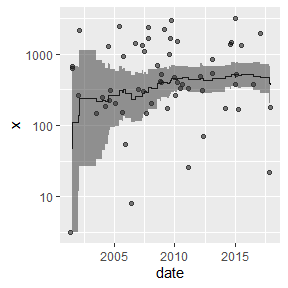### Mean

Plot the rolling 7-year mean:

Dissolved_Oxygen %>%
mutate(Station_ID = as.factor(Station_ID)) %>%
group_by(Station_ID) %>%
tbr_mean(Average_DO, Date, "years", 7, conf = 0.95, type = "perc") %>%
ggplot() +
geom_point(aes(Date, Average_DO, color = Station_ID), alpha = 0.5) +
geom_step(aes(Date, mean, color = Station_ID)) +
geom_ribbon(aes(x = Date, ymin = lwr_ci, ymax = upr_ci, fill = Station_ID), alpha = 0.5, stat = "stepribbon")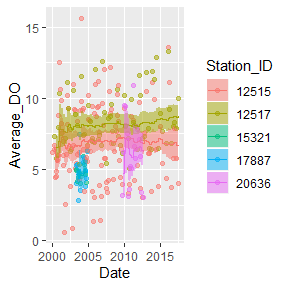### Median

Plot the rolling 7-year median:

Dissolved_Oxygen %>%
mutate(Station_ID = as.factor(Station_ID)) %>%
group_by(Station_ID) %>%
tbr_median(Average_DO, Date, "years", 7, conf = 0.95, type = "perc") %>%
ggplot() +
geom_point(aes(Date, Average_DO, color = Station_ID), alpha = 0.5) +
geom_step(aes(Date, median, color = Station_ID)) +
geom_ribbon(aes(x = Date, ymin = lwr_ci, ymax = upr_ci, fill = Station_ID), alpha = 0.5, stat = "stepribbon")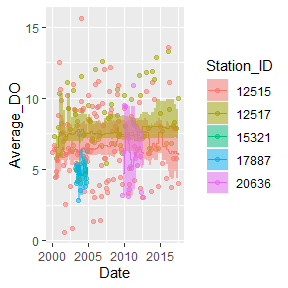### Generic functions

tbr_misc() is included to apply functions that accept a single vector of values.

For example, identify the minimum values during the previous 7 year time periods:

Dissolved_Oxygen %>%
tbr_misc(Average_DO, Date, "years", 7, func = min) %>%
ggplot() +
geom_point(aes(Date, Average_DO), alpha = 0.5) +
geom_line(aes(Date, results))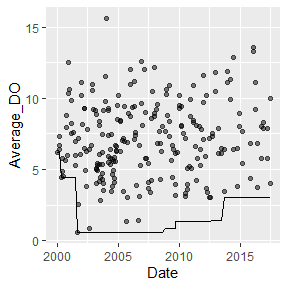### Standard Deviation

Plot the rolling 7-year SD:

Dissolved_Oxygen %>%
tbr_sd(Average_DO, Date, "years", 7) %>%
ggplot() +
geom_line(aes(Date, sd))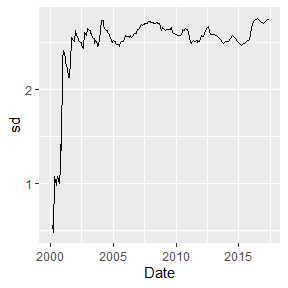### Sum

Plot the rolling 7-year sum:

Dissolved_Oxygen %>%
mutate(Station_ID = as.factor(Station_ID)) %>%
group_by(Station_ID) %>%
tbr_sum(Average_DO, Date, "years", 7) %>%
ggplot() +
geom_line(aes(Date, sum, color = Station_ID))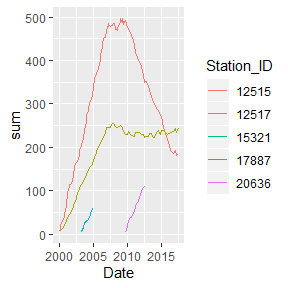## Units

Allowable character values for unit include c("years", "months", "weeks", "days", "hours", "minutes", "seconds"). Example using "minutes" and "hours":

y = 3 * sin(2 * seq(from = 0, to = 4*pi, length.out = 100)) + rnorm(100)
time = sample(seq(as.POSIXct(strptime("2017-01-01 00:01:00", "%Y-%m-%d %H:%M:%S")),
as.POSIXct(strptime("2017-01-01 23:00:00", "%Y-%m-%d %H:%M:%S")),
by = "min"), 100)

df <- data_frame(y, time)

df %>%
tbr_mean(y, time, "minutes", n = 30) %>%
ggplot() +
geom_point(aes(time, y)) +
geom_line(aes(time, mean))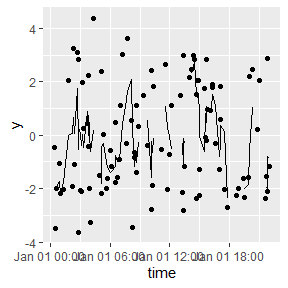df %>%
tbr_mean(y, time, "minutes", n = 60) %>%
ggplot() +
geom_point(aes(time, y)) +
geom_line(aes(time, mean))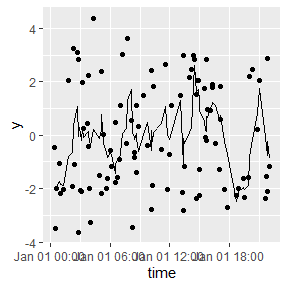df %>%
tbr_mean(y, time, "hours", n = 5) %>%
ggplot() +
geom_point(aes(time, y)) +
geom_line(aes(time, mean))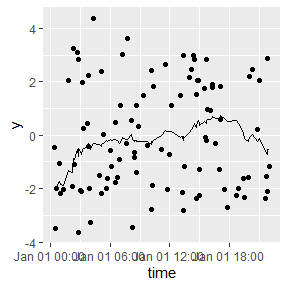## CI method

Confidence intervals in tbr_gmean, tbr_mean, and tbr_median are calculated using boot_ci. If you do not need confidence intervals, calulcation times are substantially shorter. parallel, ncores, and cl arguments are passed to boot and can improve computation times. An example using parallel processing for Windows systems is below:

library(snow)

cl <- makeCluster(4, type = "SOCK")

tbr_mean(Dissolved_Oxygen, Average_DO, Date,
"years", 5, R = 1000, conf = .95,
type = "perc", parallel = "snow",
cl = cl)

stopCluster(cl)